Question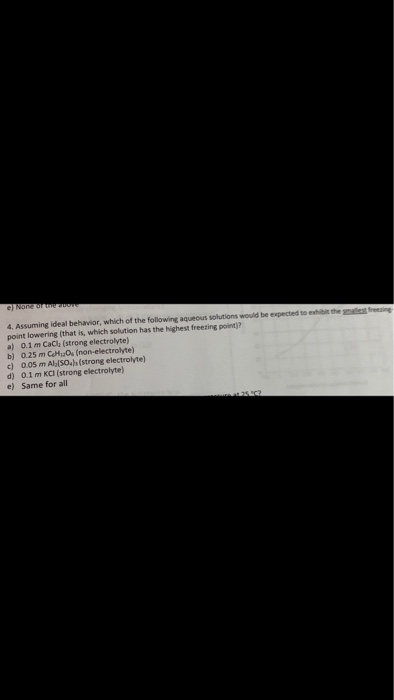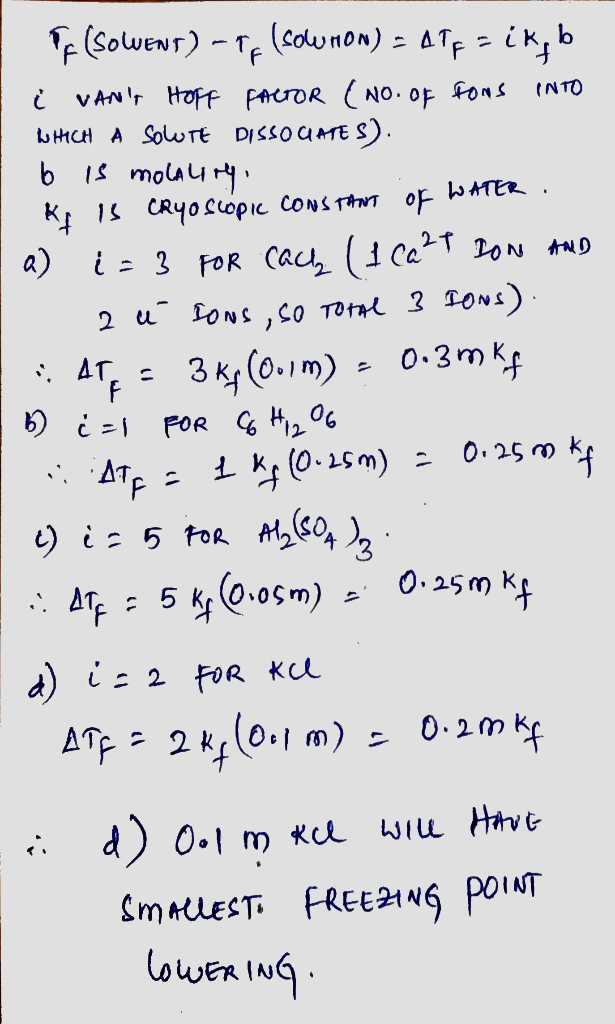#### Earn Coins

Coins can be redeemed for fabulous gifts.

Similar Homework Help Questions
• ### Which of the following ideal aqueous solutions would be predicted to have the highest freezing point?...

Which of the following ideal aqueous solutions would be predicted to have the highest freezing point? 1.00 m Na2SO4 1.00 m RbCl 0.50 m KNO3 2.00 m methanol (CH3OH)

• ### XA WinAna): Molarity: M e (mole)/Volume of solution in L), molality me molemas oletne Raoult's law:...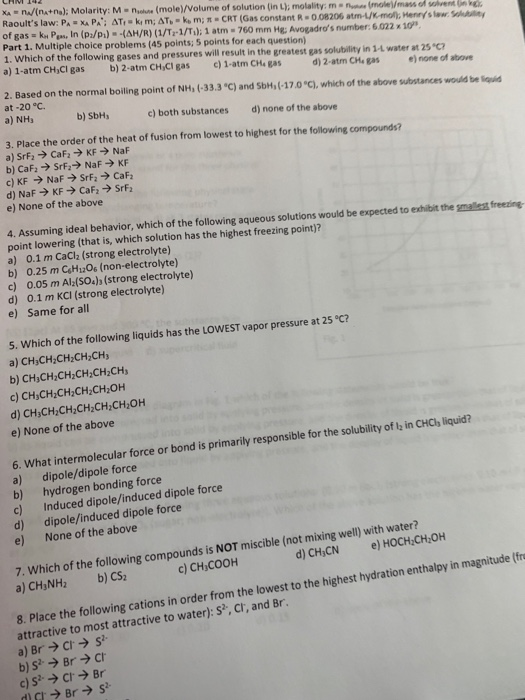XA WinAna): Molarity: M e (mole)/Volume of solution in L), molality me molemas oletne Raoult's law: PAXA PA' AT, kim; AT. - kom - CRT (Gas constant R0.08206 atm-/mol; Henry's law. of gas = k. Per In (p/p) -(AH/R) (1/T2-1/T.): 1 atm = 760 mm He: Avogadro's number: 6.022 x 10" Part 1. Multiple choice problems (45 points: 5 points for each question) 1. Which of the following gases and pressures will result in the greatest as solubility in 1...

• ### Choose the aqueous solution below with the highest freezing point. These are all solutions of nonvolatile...

Choose the aqueous solution below with the highest freezing point. These are all solutions of nonvolatile solutes and you should assume ideal van't Hoff factors where applicable. 0.25 m (NH4)3PO4 0.35 m K2CO3 0.45 m KCN

• ### 12. Which of the following aqueous solutions would give the lowest freezing point? You must justify...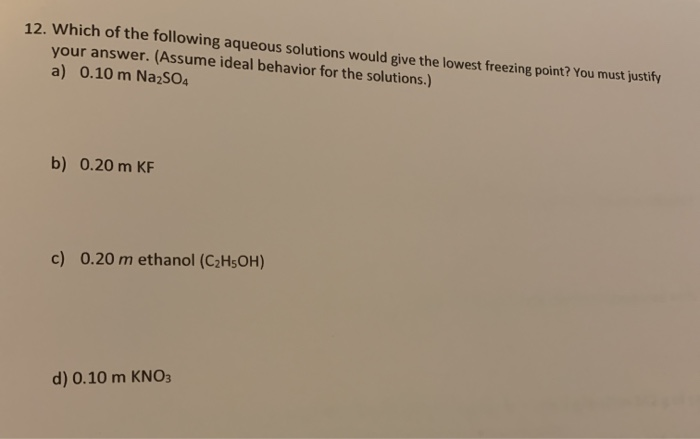12. Which of the following aqueous solutions would give the lowest freezing point? You must justify your answer. (Assume ideal behavior for the solutions.) a) 0.10 m Na2SO4 b) 0.20 m KF c) 0.20 m ethanol (C2H5OH) d) 0.10 m KNO3

• ### Choose the aqueous solution below with the highest freezing point. These are all solutions of nonvolatile...

Choose the aqueous solution below with the highest freezing point. These are all solutions of nonvolatile solutes and you should assume ideal van't Hoff factors where applicable. Choose the aqueous solution below with the highest freezing point. These are all solutions of nonvolatile solutes and you should assume ideal van't Hoff factors where applicable. 1. 0.200 m HOCH2CH2OH 2. 0.200 m Na3PO3 3. 0.200 m Ba(NO3)2 4. 0.200 m Mg(ClO4)2 5. These all have the same freezing point. (please explain...

• ### Which of the following 0.100 m aqueous solutions would have the lowest melting point? answer choices:...

Which of the following 0.100 m aqueous solutions would have the lowest melting point? answer choices: A. KClO4 B. sucrose (non-electrolyte) c. Ca(ClO4)2 d. Al(ClO4)3        e. NaCl Ca(ClO4)2 Al(ClO4)3        NaCl

• ### Match the following aqueous solutions with the appropriate letter from the column on the right. 1....

Match the following aqueous solutions with the appropriate letter from the column on the right. 1. 0.16 m NiBr2 A. Lowest freezing point 2. 0.15 m Mg(NO3)2 B. Second lowest freezing point 3. 0.20 m MnSO4 C. Third lowest freezing point 4. 0.42 m Sucrose(nonelectrolyte) D. Highest freezing point 2.The boiling point of diethyl ether, CH3CH2OCH2CH3, is 34.500 °C at 1 atmosphere. Kb(diethyl ether) = 2.02 °C/m In a laboratory experiment, students synthesized a new compound and found that when...

• ### 1) Which ONE of the following aqueous solutions contains the lowest concentration of cations, assuming 100%...

1) Which ONE of the following aqueous solutions contains the lowest concentration of cations, assuming 100% dissolution and ionization? (A) 0.5 M sodium phosphate (B) 1.0 M potassium nitrate (C) 2.0 M magnesium sulfate (D) 0.75 M calcium chloride (E) 1.5 M hydrogen chloride 2) The highest mass of SnS will dissolve in 1.0 L of an aqueous solution of: (A) 0.1 M MgS (B) 0.1 M (NH4)2S (C) 0.1 M Sn(NO3)2 (D) 0.1 M Na2S (E) 0.1 M CaCl2

• ### Which of the following aqueous solutions has the highest boiling point? Kb for water is 0.52°C/m....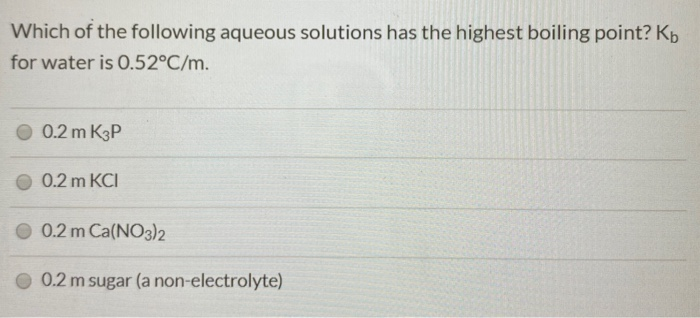Which of the following aqueous solutions has the highest boiling point? Kb for water is 0.52°C/m. 0.2 m K3P 0.2 m KCI 0.2 m Ca(NO3)2 0.2 m sugar (a non-electrolyte)

• ### Which of the following aqueous solutions has the lowest freezing point? The Kf of water is...

Which of the following aqueous solutions has the lowest freezing point? The Kf of water is 1.86 kg.oC/mol. a. 0.8 m CH3OH (methanol) b. 0.4 m NaCl c. 0.3 m MgBr2 d. 0.2 m AlF3 e. 0.25 m Ca(NO3)2 Calculate the freezing point ONLY for the solution you marked as your answer above.